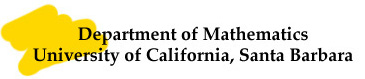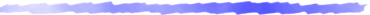### Advanced Linear Algebra

####Office Hours: MW 11-12noon, F 12:30-1:30pm in SH 6511##The \$25Billion Eigenvector

HW #1 (due 10/5)
Batch 1:
1) Verify that <x, y>_k (you decide what `k' stands for :))=nx_1y_1+(n-1)x_2y_2 + ...  + x_ny_n defines an inner product on R^n. Then compute the norm of
u=(a_1, a_2, ..., a_n).
2) Given real numbers a_ij, i, j=1, 2, define <x, y>=a_11 x_1 y_1 + a_12 x_1 y_2 + a_21 x_2 y_1 + a_22 x_2 y_2, determine the conditions that make <x, y>
an inner product on R^2.
3) Given an inner product on R^2, compute ||x-y||. Hence use the cosine law to deduce that <x, y>=||x|| ||y|| cos \theta, with \theta =the angle between x and y, when <x, y> is the dot product.

Batch 2:
p122: 2, 6.

Batch 3:
p122: 3, 4, 5.

• Solutions

• HW #2 (due 10/12)
Batch 1:
p122: 7, 8(hint: given ||v||, how would you define <u, v>? you need to check 1). positivity; 2) linear in first slot; 3) symmetry/conjugate symmetry. You can skip the linearity), 9, 13.

Batch 2:
1) For V=R^3, <x, y>=dot product, v_1=(1, 0, 1), v_2=(0, 1, 1), v_3=(1, 3, 3), apply the Gram-Schmidt to obtain an orthonormal basis e_1, e_2, e_3. Then write
v=(1, 1, 2)  in terms of e_1, e_2, e_3.
2) For V=R^2, <x, y>=2 x_1 y_1 - x_1 y_2 - x_2 y_1 + 2 x_2 y_2, apply G-S to v_1=(1, 0), v_2=(0, 1) to obtain an orthonormal basis e_1, e_2.
p123: 10, 11.

Batch 3:
1). Prove that U^\perp is always a subspace of V.
2). For V=R^3, <x, y>=x_1y_1+x_2y_2-x_1y_3-x_3y_1+4x_3y_3, extend the orthonormal list e_1=(1,0, 0), e_2=(0, 1, 0) to an othonormal basis.
p124: 16.

• Solutions

• HW #3 (due 10/19)
Batch 1:
p124: 21, 22, 23.

Batch 2:
1) Let V be an inner product space (real or complex) and e_1, e_2, ..., e_n an orthonormal basis. Show that for any u, v in V
<u, v>=<u, e_1>\overline{<v, e_1>} + ... + <u, e_n>\overline{<v, e_n>} (Here \overline{ } means the complex conjugate; for real vector space, it does nothing)
What happens when u=v?
p125: 27, 28.

• Solutions

• Solutions to Midterm I

• HW #4 (due 10/26)
Batch 1:
p124: 19, 29, 30.

Batch 2:
1). Verify a) (T_1+T_2)^*=T_1^* + T_2^*; b) (aT)^*=\bar{a} T^*; (ST)^*=T^*S^*.
p125: 30, 31.

Batch 3:
p125: 24.

• Solutions

• HW #5 (due 11/2)
Batch 1:
p158: 1,  2, 3(a).

Batch 2:
p159: 8, 15. Plus
1). Consider T: R^2 -----> R^2 given by T(x)=Ax where A = matrix( 0, 1 \\ -1, 0). Show that T^2+I=0. Compare this with Lemma 7.11.
2). Consider T: R^2 -----> R^2 given by T(x)=Ax where A = matrix( 1, 1 \\ 0, 1). Show that U={x_2=0} is invariant under T but U^{\perp} is NOT.

Batch 3:
1). Determine if the following matrices can be diagonalized. A_1=matrix( 1, 2, 3, 4, 5\\ 2, 2, 3, 4, 5\\ 3, 3, 3, 4, 5\\4, 4, 4, 4, 5\\ 5, 5, 5, 5, 5)
A_2= matrix(2, 0, 0, 0, 0\\1, 2, 0, 0, 0\\2, 1, 2, 0, 0\\1, 2, 1, 2, 0\\2, 1, 2, 1, 2).
2). Let A be a symmetric real matrix and \alpha, \beta real numbers such that  A^2+ \alpha A + \beta I=0.
(a). Show that \alpha^2 >= 4\beta.
(b). Show that there are real numbers \lambda_1, \lambda_2 such that (A- \lambda_1 I)(A- \lambda_2 I)=0.
(c). Deduce that one of the \lambda_1, \lambda_2 is an eigenvalue of A.

• Solutions

• HW #6 (due 11/9)
Batch 1:
1). Let C^2 be given the inner product <z, w>=4z_1 \bar{w_1} + 2z_1 \bar{w_2}+ 2z_2 \bar{w_1}+ 2 z_2 \bar{w_2} and T: C^2 ---> C^2 be given by T(z)=Az
where A = matrix( 2, 3/2 \\ 0, -1). Determine if T is self adjoint (with respect to this given inner product).
2). Same for A = matrix( 1, 0 \\ 0, 2).
3). Given a complex vector space V and a linear map T: V ---> V, define T_1=1/2(T+T*), T_2=1/(2i)(T-T*).
(a). Show that both T_1, T_2 are self adjoint.
(b). Show that T is normal if and only if T_1T_2=T_2T_1.

Batch 2:
1). Verify that for any linear map T on a complex inner product space V, <Tu, v>=1/4[ <T(u+v), u+v> - <T(u-v), u-v> + i<T(u+iv), u+iv> - i<T(u-iv), u-iv>].
2). Let V be an inner product space (real or complex), T_1, T_2 are linear maps on V such that T_1T_2=T_2T_1. Show that null(T_1-\lambda I) is invariant under T_2.
3). Same as in 2). In addition, T_1, T_2 are self adjoint. For simplicity, assume that T_1 has only two distinct eigenvalues \lambda_1, \lambda_2.
(a) Use Corollary 7.14 to deduce that V=null(T_1-\lambda_1 I) \oplus null(T_1-\lambda_2 I).
(b) Use 2) to get T_2: null(T_1-\lambda_1 I) ---> null(T_1-\lambda_1 I) and T_2: null(T_1-\lambda_2 I) ---> null(T_1-\lambda_2 I). Hence obtain an o.n. basis
e_1, ... , e_k of null(T_1-\lambda_1 I), and o.n. basis \tilde{e}_1, ..., \tilde{e}_l of null(T_1-\lambda_2 I).
(c) Conclude that there is an o.n. basis of V which are eigenvectors of both T_1 and T_2.

Batch 3:
p158: 6; plus
1). Let V be an real inner product space and T: V ---> V self adjoint. Derive a formula for <Tu, v> in terms of <T(u+v), u+v> and <T(u-v), u-v>. Hence deduce that
<Tv, v>=0 for all v in V implies that T=0. Give an example when this fails if T is not self adjoint.

• Solutions

• HW #7 (due 11/16)
Batch 1:
1). Let T be a linear map on V and U a subspace of V.
(a) Show that U is invariant under T if and only if there is a basis v_1, ... , v_m of U such that Tv_1, ... , Tv_m are all in U;
(b) Conclude that U=span(u_1, ...,  u_k) where each u_1, ..., u_k is an eigenvector of T, is invariant under T;
(c) What is a one dimensional invariant subspace?
2). Let V be a complex inner product space of dim 3, T is a normal map on V and U an invariant subspace of T of dim 2.  Show that U^{\perp} is also invariant.
3). Same as above but dim V=4.

Batch 2:
p159: 17, 18; Plus
1). Recall that for a linear map T on a complex inner product space, T=T_1+iT_2, with T_1=1/2(T+T*) and T_2=1/(2i)(T-T*) both self adjoint. Moreover, T is normal if and only if T_1T_2=T_2T_1.
(a) If u is an eigenvector of both T_1, T_2, show that u is also an eigenvector of T.
(b) Give another proof of the Complex Spectral Theorem II by using the Complex Spectral Theorem I and HW#6.

• Solutions

• Solutions to Midterm II

• HW #8 (due 12/3, postponed to 12/5)
Batch 1:
p188: 3, plus
1). Find all generalized eigenvectors of T: C^4 -----> C^4 given by T(x)=Ax where A = matrix( 2, 2, 2, 2 \\  0, 2, 2, 2 \\ 0, 0, 2, 2 \\ 0, 0, 0, 2).
2) Use the definition of generalized eigenvectors to show that the set of all generalized eigenvectors with eigenvalue \lambda is a subspace.

Batch 2:
p188: 5, 6, 7.

Batch 3:
1). Let U be a complex vector space and N: U ---> U a nilpotent operator. Put V=range N. Assume that there are vectors v_1, v_2, v_3 in  V such that
v_1, Nv_1, v_2, Nv_2, v_3, Nv_3, N^2v_3 gives a basis of V and Nv_1, Nv_2, N^2v_3 form a basis of null N \cap V.
(a) Show that there are vectors u_1, u_2, u_3, ... , u_k (k>=3) such that u_1, Nu_1, N^2u_1, u_2, Nu_2, N^2u_2,  u_3, ..., N^3u_3, u_4, ... , u_k form a basis of U
and N^2u_1, N^2u_2, N^3u_3, u_4, ... , u_k gives a basis of null N.
(b) Find the Jordan form of N in this basis.

• Solutions

• dai@math.ucsb.edu